## 【Flag】机器学习100天-[第2天]-线性回归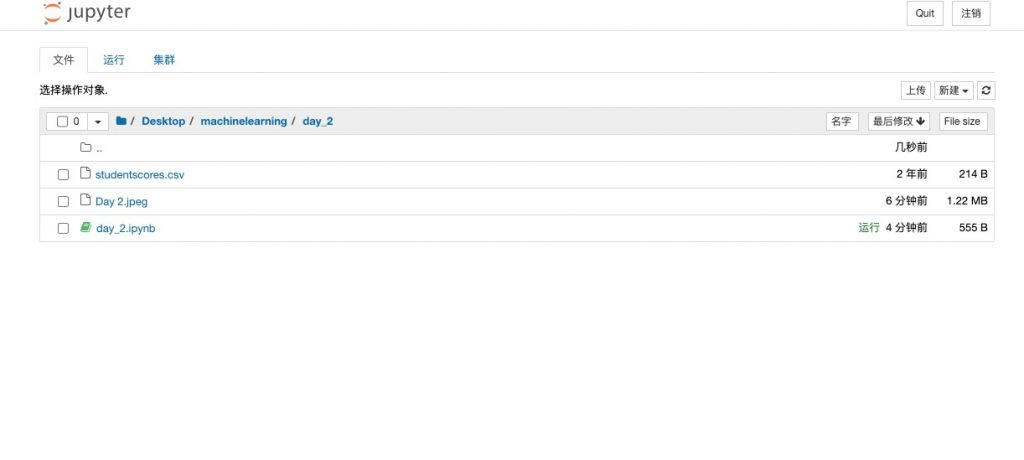## 导入数据集并切分训练集和测试集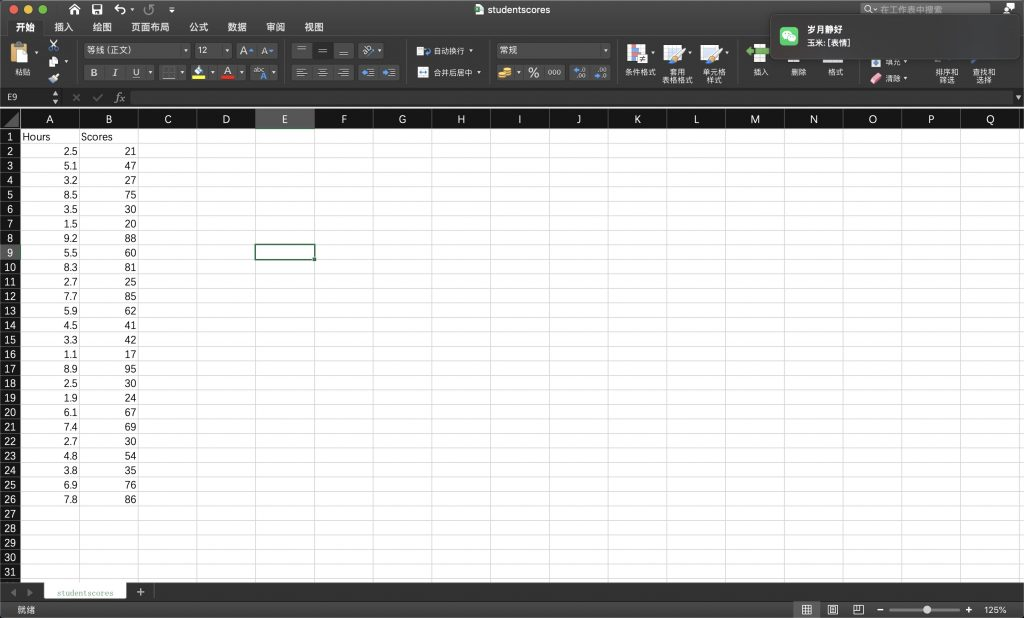25 x 2 数据集

import pandas as pd
import numpy as np
import matplotlib.pyplot as plt

X = dataset.iloc[ : , : 1 ].values
Y = dataset.iloc[ : , 1 ].values

from sklearn.model_selection import train_test_split
X_train, X_test, Y_train, Y_test = train_test_split( X, Y, test_size = 1/4, random_state = 0)

print("X_train:", X_train)
print("X_test:", X_test)
print("Y_train:", Y_train)
print("Y_test:", Y_test)## 使用训练集X_train, Y_train 进行线性回归

from sklearn.linear_model import LinearRegression
regressor = LinearRegression()
regressor = regressor.fit(X_train, Y_train)

print(regressor.coef_)

## 数据可视化

### 使用训练集X_train, Y_train 数据查看回归方程拟合效果

plt.scatter(X_train , Y_train, color = 'red')
plt.plot(X_train , regressor.predict(X_train), color ='blue')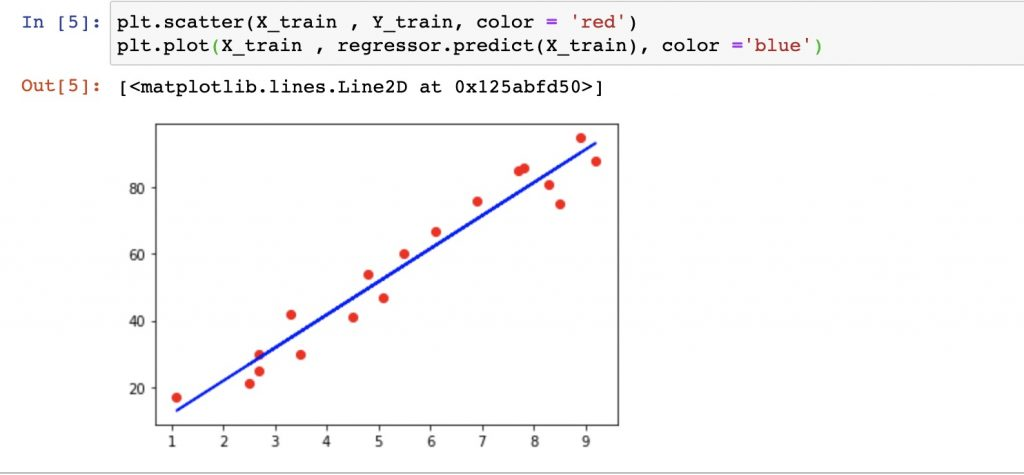### 使用测试集Y_test, X_test 数据检验回归方程regressor

Y_pred = regressor.predict(X_test)
print(Y_pred)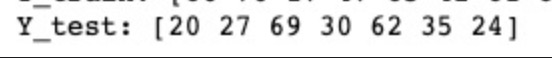regressor计算值：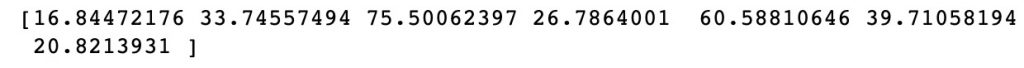plt.scatter(X_test , Y_test, color = 'red')
# plt.plot(X_test , regressor.predict(X_test), color ='blue')
print("==="*5)
plt.plot(X_test , Y_pred, color ='blue')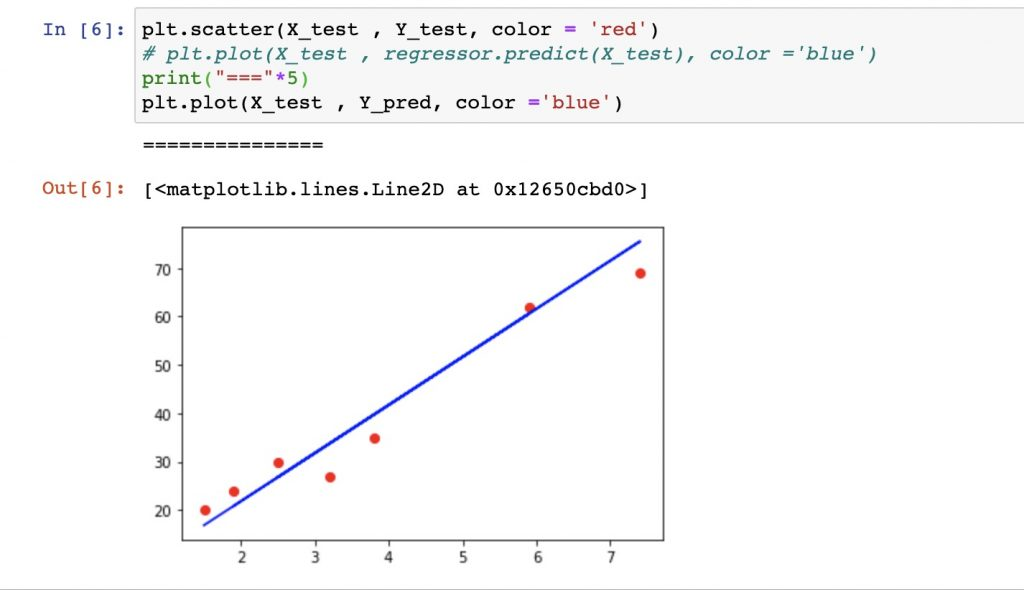## 补充内容

### 线性回归原理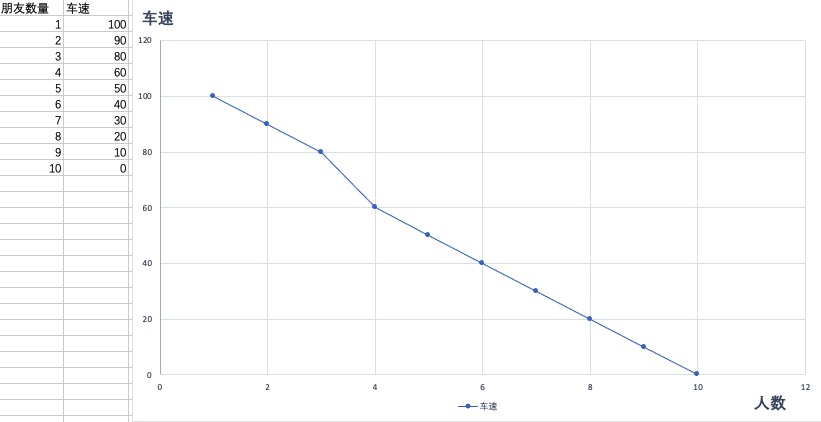### 一元线性回归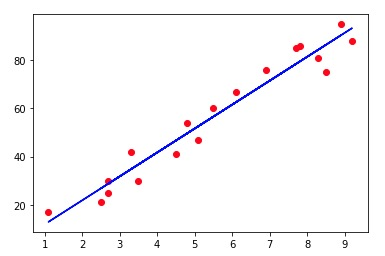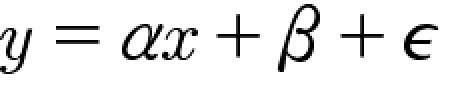### 损失厌恶和损失函数

#### 基本逻辑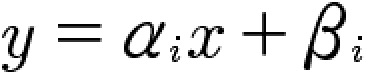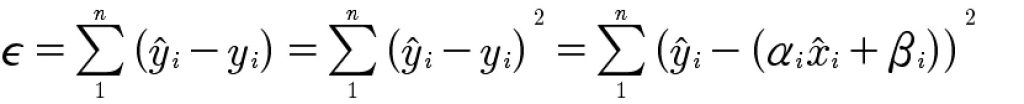#### 损失函数最小值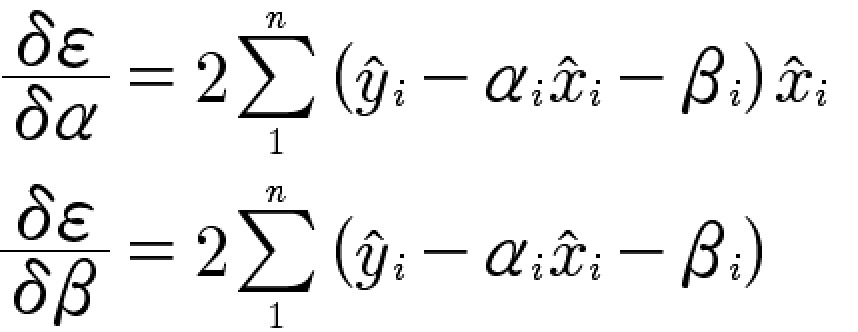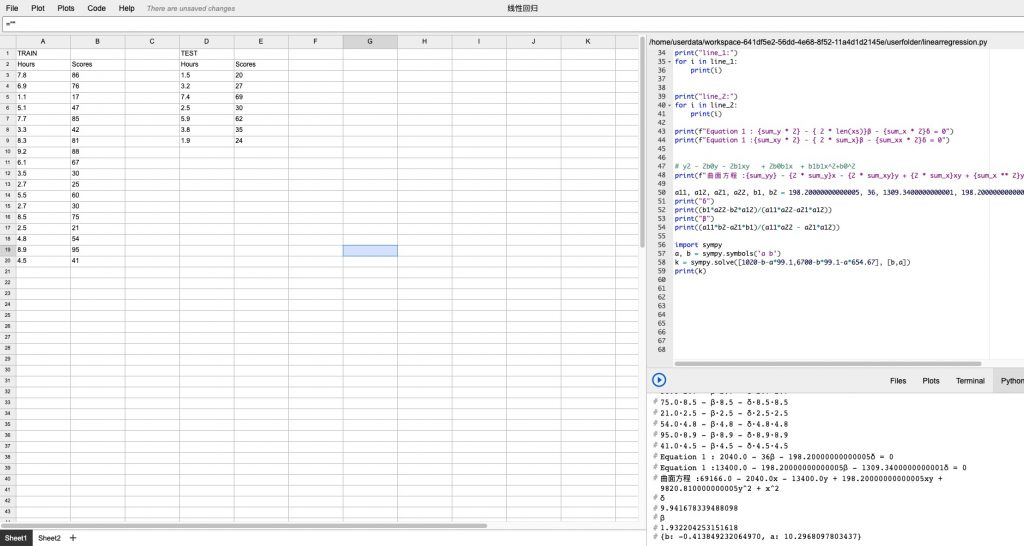Gridstudio

Equation 1 : 2040.0 – 36β – 198.20000000000005α = 0
Equation 2 :13400.0 – 198.20000000000005β – 1309.3400000000001α = 0

α: -0.413849232064970；β: 10.2968097803437

gridstudio 及分析代码下载：

gridstudio：github

文件名称：manual_linear_process.py
文件大小：1KB
下载声明：本站部分资源来自于网络收集，若侵犯了你的隐私或版权，请及时联系我们删除有关信息。

## 笔记下载

文件名称：第二天数据集及笔记文件
文件大小：1MB
下载声明：本站部分资源来自于网络收集，若侵犯了你的隐私或版权，请及时联系我们删除有关信息。
下载地址：day_2